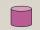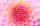Discriminant

Determine the discriminant of the equation:Result

D =  -167

Solution:Leave us a comment of example and its solution (i.e. if it is still somewhat unclear...):Be the first to comment!To solve this example are needed these knowledge from mathematics:

Looking for help with calculating roots of a quadratic equation?

Next similar examples:

1. Variations 4/2Determine the number of items when the count of variations of fourth class without repeating is 600 times larger than the count of variations of second class without repetition.
2. AS sequenceIn an arithmetic sequence is given the difference d = -3 and a71 = 455. a) Determine the value of a62 b) Determine the sum of 71 members.
3. RootsDetermine the quadratic equation absolute coefficient q, that the equation has a real double root and the root x calculate: ?
4. EquationEquation ? has one root x1 = 8. Determine the coefficient b and the second root x2.Find the roots of the quadratic equation: 3x2-4x + (-4) = 0.Which of the points belong function f:y= 2x2- 3x + 1 : A(-2, 15) B (3,10) C (1,4)
7. CombinationsHow many elements can form six times more combinations fourth class than combination of the second class?
8. Cylinder diameterThe surface of the cylinder is 149 cm2. The cylinder height is 6 cm. What is the diameter of this cylinder?
9. 2nd class combinationsFrom how many elements you can create 4560 combinations of the second class?
10. Sequence 3Write the first 5 members of an arithmetic sequence: a4=-35, a11=-105.
11. CombinationsFrom how many elements we can create 990 combinations 2nd class without repeating?
12. Solve 3Solve quadratic equation: (6n+1) (4n-1) = 3n2
13. Variation equationSolve combinatorics equation: V(2, x+8)=72
14. VariableFind variable P: PP plus P x P plus P = 160
15. Complex number coordinatesWhich coordinates show the location of -2+3i
16. Calculation of CNCalculate: ?
17. Theorem proveWe want to prove the sentence: If the natural number n is divisible by six, then n is divisible by three. From what assumption we started?